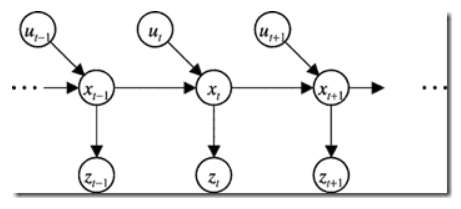数学表达

• 系统任意时刻可以用有限个可能状态之一来描述
• 系统无后效性，即某阶段的状态一旦确定，则此后过程的演变不再受此前各种状态及决策的影响
无后效性(附录有详细描述)

条件一 …… 概率向量(状态向量)

${X}^{\left(n\right)}=\left({x}_{1}^{\left(n\right)}\phantom{\rule{1em}{0ex}}{x}_{2}^{\left(n\right)}\phantom{\rule{1em}{0ex}}\dots \phantom{\rule{1em}{0ex}}{x}_{k}^{\left(n\right)}{\right)}^{T}$$X^{(n)} = (x_{1}^{(n)} \quad x_{2}^{(n)} \quad \dots \quad x_{k}^{(n)})^{T}$

• 概率向量的每个元素都是概率，并且元素之和为1。
• k是系统的可能状态数。
• ${x}_{i}^{\left(n\right)}$$x_{i}^{(n)}$表示第n次观测时第i个状态的概率

${X}^{0}$$X^{0}$被称为马尔科夫链的初始状态

条件二 …… 转移概率矩阵

$P=\left(\begin{array}{ccccccc}{p}_{11}& & p12& & \dots & & p1k\\ {p}_{21}& & p22& & \dots & & p2k\\ ⋮& & ⋮& & \phantom{\rule{1em}{0ex}}& & ⋮\\ {p}_{k1}& & pk2& & \dots & & pkk\end{array}\right)$$P = \begin{pmatrix} p_{11} && p{12} && \dots && p{1k} \\ p_{21} && p{22} && \dots && p{2k} \\ \vdots && \vdots && \quad && \vdots \\ p_{k1} && p{k2} && \dots && p{kk} \\ \end{pmatrix}$

• ${p}_{ij}\left(i,j=1,2,\dots ,k\right)$$p_{ij} (i,j=1,2,\dots,k)$表示这次观测时状态为j，现在观测是状态为i的概率
• P矩阵元素非负
• 每一列的元素之和都为1

$\phantom{\rule{2em}{0ex}}\phantom{\rule{2em}{0ex}}\phantom{\rule{2em}{0ex}}\phantom{\rule{2em}{0ex}}{X}^{\left(n\right)}={P}^{n}{X}^{\left(0\right)}$$\qquad \qquad \qquad \qquad X^{(n)} = P^{n}X^{(0)}$

例子

 归还\借出 1 2 3 1 0.5 0.3 0.3 2 0.2 0.1 0.6 3 0.3 0.6 0.1

$P=\left(\begin{array}{ccccc}0.5& & 0.3& & 0.3\\ 0.2& & 0.1& & 0.6\\ 0.3& & 0.6& & 0.1\end{array}\right)$$P = \begin{pmatrix} 0.5 && 0.3 && 0.3 \\ 0.2 && 0.1 && 0.6 \\ 0.3 && 0.6 && 0.1 \\ \end{pmatrix}$

$P{X}^{\left(0\right)}={X}^{\left(1\right)}=\left(0.3,0.1,0.6{\right)}^{T}$$PX^{(0)} = X^{(1)} = (0.3, 0.1, 0.6)^{T}$
${X}^{\left(2\right)}=\left(0.35,0.43,0.21{\right)}^{T}$$X^{(2)} = (0.35, 0.43,0.21)^{T}$
${X}^{\left(3\right)}=\left(0.324,0.239,0.384{\right)}^{T}$$X^{(3)} = (0.324,0.239,0.384)^{T}$

附录

1. 马尔科夫假设的概率理解

t时刻的状态和t-1时刻和t时刻的动作决定。t时刻的观测仅仅同t时刻的状态相关$P\left({z}_{t}|{x}_{0:t},{z}_{z:t},{u}_{1:t}\right)=P\left({z}_{t}|{x}_{t}\right)\phantom{\rule{0ex}{0ex}}P\left({x}_{t}|{x}_{1:t-1},{z}_{1:t},{u}_{1:t}\right)=P\left({x}_{t}|{x}_{t-1},{u}_{t}\right)$